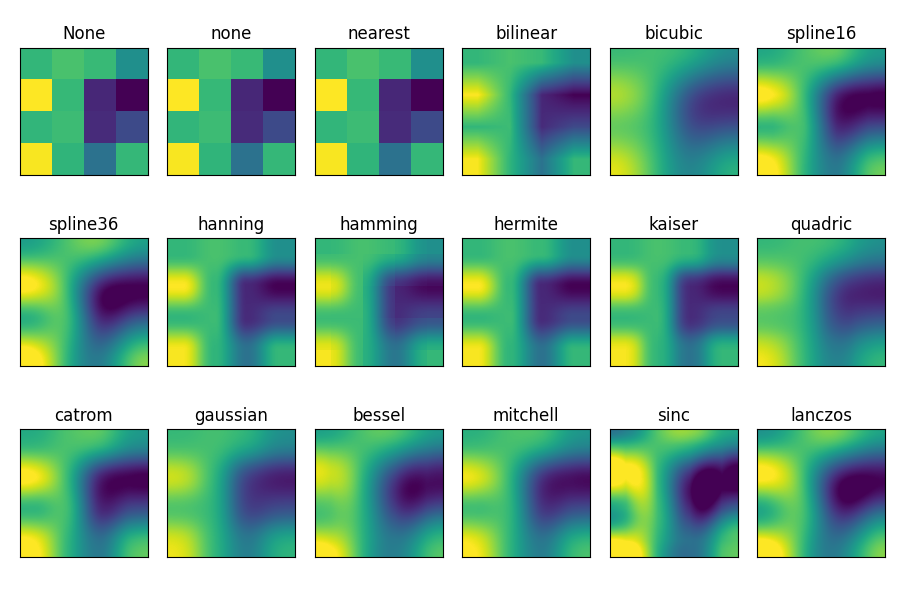# Interpolations for imshow¶

This example displays the difference between interpolation methods for imshow().

If interpolation is None, it defaults to the rcParams["image.interpolation"] (default: 'antialiased'). If the interpolation is 'none', then no interpolation is performed for the Agg, ps and pdf backends. Other backends will default to 'antialiased'.

For the Agg, ps and pdf backends, interpolation = 'none' works well when a big image is scaled down, while interpolation = 'nearest' works well when a small image is scaled up.

See Image antialiasing for a discussion on the default interpolation="antialiased" option.

import matplotlib.pyplot as plt
import numpy as np

methods = [None, 'none', 'nearest', 'bilinear', 'bicubic', 'spline16',
'spline36', 'hanning', 'hamming', 'hermite', 'kaiser', 'quadric',
'catrom', 'gaussian', 'bessel', 'mitchell', 'sinc', 'lanczos']

# Fixing random state for reproducibility
np.random.seed(19680801)

grid = np.random.rand(4, 4)

fig, axs = plt.subplots(nrows=3, ncols=6, figsize=(9, 6),
subplot_kw={'xticks': [], 'yticks': []})

for ax, interp_method in zip(axs.flat, methods):
ax.imshow(grid, interpolation=interp_method, cmap='viridis')
ax.set_title(str(interp_method))

plt.tight_layout()
plt.show()